# PyTorch使用记录022019年6月14日07:59:21

13325字阅读44分25秒

## Pytorch动态调整学习率

### 基本的动态调节

1. from torch.optim import lr_scheduler
2. test_optimizer = optim.SGD([torch.randn(1, requires_grad=True)], lr=1e-3)
3. exp_lr_scheduler = optim.lr_scheduler.StepLR(test_optimizer, step_size=3, gamma=0.5)
4. for epoch in range(1, 12):
5.     exp_lr_scheduler.step() # 要每一个epoch都要使用
6.     print('Epoch {}, lr {}'.format(
7.         epoch, test_optimizer.param_groups['lr']))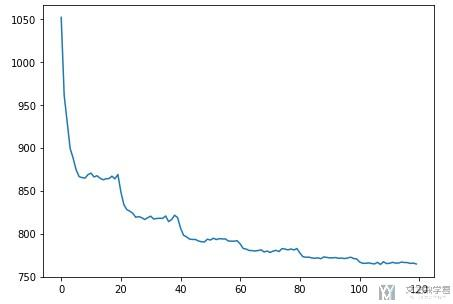### 不同的调节方法结合与CosineAnnealingLR介绍

1. test_optimizer.param_groups['initial_lr'] = test_optimizer.param_groups['lr'] # 需要修改optimizer的initial lr

1. from torch.optim import lr_scheduler
2. test_optimizer = torch.optim.SGD([torch.randn(1, requires_grad=True)], lr=1e-3)
3. exp_lr_scheduler = torch.optim.lr_scheduler.StepLR(test_optimizer, step_size=3, gamma=0.5)
4. for epoch in range(1, 12):
5.     exp_lr_scheduler.step() # 要每一个epoch都要使用
6.     print('Epoch {}, lr {}'.format(
7.         epoch, test_optimizer.param_groups['lr']))
8. print('='*10)
9. test_optimizer.param_groups['initial_lr'] = test_optimizer.param_groups['lr'] # 需要修改optimizer的initial lr
10. cos_lr_scheduler = torch.optim.lr_scheduler.CosineAnnealingLR(test_optimizer, T_max = 3, eta_min=0.00001)
11. for epoch in range(12, 24):
12.     cos_lr_scheduler.step() # 要每一个epoch都要使用
13.     print('Epoch {}, lr {}'.format(
14.         epoch, test_optimizer.param_groups['lr']))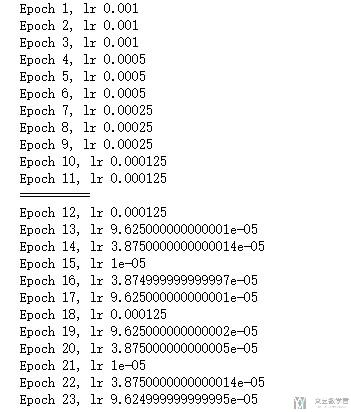### 调节算法MultiStepLR介绍

1. >>> scheduler = MultiStepLR(optimizer, milestones=[30,80], gamma=0.1)
2. >>> # Assuming optimizer uses lr = 0.05 for all groups
3. >>> # lr = 0.05     if epoch < 30
4. >>> # lr = 0.005    if 30 <= epoch < 80
5. >>> # lr = 0.0005   if epoch >= 80

## Pytorch中图片数据集处理方式

Pytorch中有比较简单的图片数据集的处理方式, 我们可以使用ImageFolder来完成图片数据集的导入. 下面我们来看一个简单的例子.

1. trans = transforms.Compose([
2.     transforms.Resize(64),
3.     transforms.ToTensor(),
4.     transforms.Normalize((0.5, 0.5, 0.5), (0.5, 0.5, 0.5))
5. ])
6. # 注意这里文件夹的路径
7. dataset = datasets.ImageFolder('./data', transform=trans) # 数据路径
9.                                      batch_size=16, # 批量大小
10.                                      shuffle=True# 乱序
11.                                      num_workers=2 # 多进程
12.                                      )

1. root/dog/xxx.png
2. root/dog/xxy.png
3. root/dog/xxz.png
4. root/cat/123.png
5. root/cat/nsdf3.png
6. root/cat/asd932_.png

## Pytorch中下划线的含义

• First of all on the PyTorch sites it is recommended to not use in-place operations in most cases. Unless working under heavy memory pressure it is more efficient in most cases to not use in-place operations.
• Secondly there can be problems calculating the gradients when using in-place operations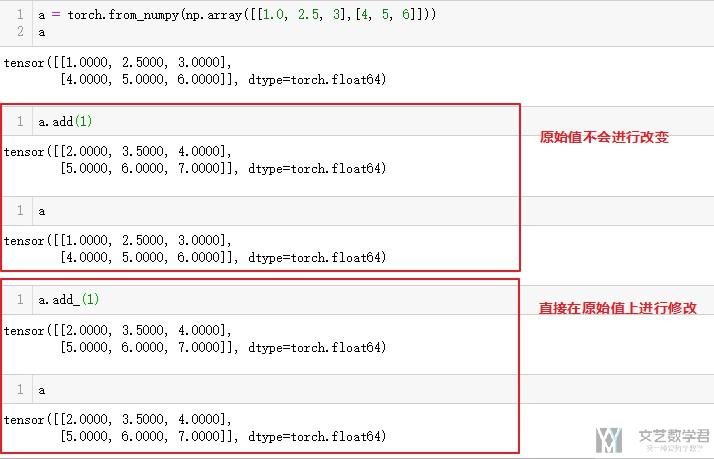## Pytorch Tensor get the index of specific value(tensor获得指定值的index)

1. test = torch.Tensor([1,2,3,1,2,3,1,2,3])

1. (test==1).nonzero().squeeze()
2. """
3. tensor([0, 3, 6])
4. """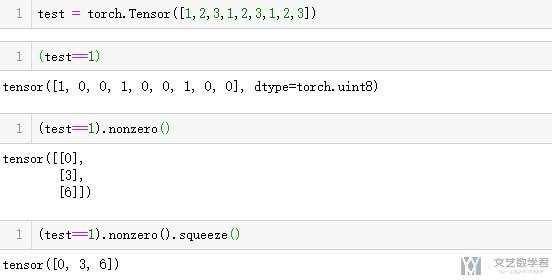## Pytorch中tensor的拼接

### torch.cat测试

torch.cat可以用来合并两个tensor向量。我们下面简单举一个例子，这个是合并一维的向量.

1. a = torch.tensor([])
2. b = torch.tensor([1,2])
3. c = torch.tensor([3,4,5])
4. a = torch.cat((a,b))
5. a = torch.cat((a,c))
6. print(a)
7. # >>> tensor([1., 2., 3., 4., 5.])

1. # torch.cat测试
2. x_test = torch.tensor([[[0,1,0]],[[1,0,1]]])
3. y_test = torch.tensor([[[2,2,2]],[[3,3,3]]])
4. print(x_test.shape)
5. print('===')
6. print(torch.cat((x_test, y_test), dim=1))
7. print(torch.cat((x_test, y_test), dim=1).shape)
8. print('===')
9. print(torch.cat((x_test, y_test), dim=0))
10. print(torch.cat((x_test, y_test), dim=0).shape)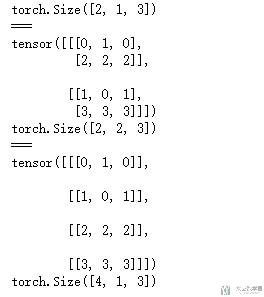### torch.stack测试

1. torch.stack((x_test, y_test)).shape

1. torch.stack(([dataset[i] for i in range(36)])).shape

## torch.norm的简单使用说明

torch.norm是用来计算范数的, 我们简单来讲解一下用法.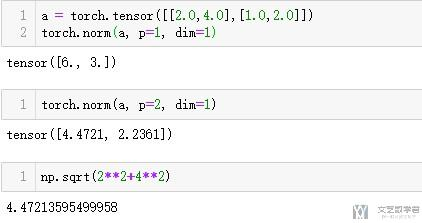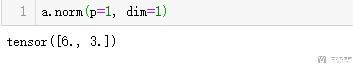## transform.Normalize()的简单介绍

1. transform = transforms.Compose([
2.                 transforms.ToTensor(),
3.                 transforms.Normalize(mean=[0.5], std=[0.5])])

Normalize是进行下面的操作:  image = (image - mean) / std

1. def denorm(x):
2.     out = (x + 1) / 2
3.     return out.clamp(0, 1)

## torch.gather使用

gather的主要用法如下图所示(这里和实际有一点不一样的地方, 这里下标是从1开始的, 实际上下标应该是从0开始的, 后面看代码的时候会有看到).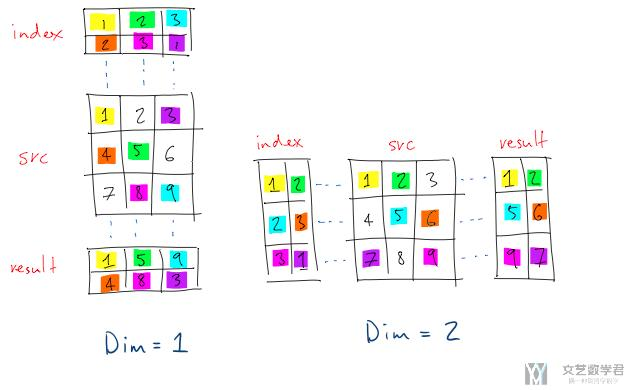1. src = torch.tensor([[1,2,3],[4,5,6],[7,8,9]]).float()
2. indexV = torch.tensor([[1,2,0],[2,0,1],[1,2,0]]).long()
3. # dim=0测试
4. torch.gather(input=src, dim=0, index=indexV)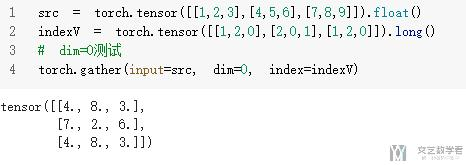dim=1代表是行, 也就是上面的dim=2的对应方式, 可以自行比较一下.

1. src = torch.tensor([[1,2,3],[4,5,6],[7,8,9]]).float()
2. indexV = torch.tensor([[1,2,0],[2,0,1],[1,2,0]]).long()
3. # dim=1测试
4. torch.gather(input=src, dim=1, index=indexV)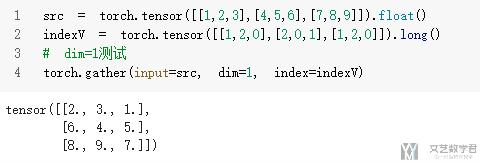## torch中常见的计算

### torch.dot-Pytroch中向量与向量乘法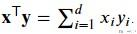1. x = torch.arange(4, dtype=torch.float32)
2. y = torch.ones(4, dtype = torch.float32)
3. x, y, torch.dot(x, y)
4. """
5. (tensor([0., 1., 2., 3.]), tensor([1., 1., 1., 1.]), tensor(6.))
6. """

### torch.mv-Pytorch中矩阵与向量乘法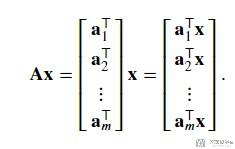1. x = torch.arange(4, dtype=torch.float32)
2. A = torch.arange(20, dtype=torch.float32).reshape(5, 4)
3. torch.mv(A, x)
4. """
5. tensor([ 14.,  38.,  62.,  86., 110.])
6. """

### torch.mm-Pytorch中的矩阵乘法

1. a = torch.from_numpy(np.array([[0,1,0],[1,0,1]]))
2. b = torch.from_numpy(np.array([[1,4],[2,5],[3,6]]))

1. torch.mm(a,b)
2. """
3. tensor([[ 2,  5],
4.         [ 4, 10]], dtype=torch.int32)
5. """

### torch.mul--Pytorch中对应位相乘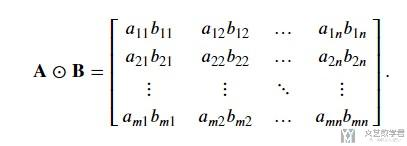1. a = torch.from_numpy(np.array([[0,1,0],[1,0,1]]))
2. b = torch.from_numpy(np.array([[1,2,3],[4,5,6]]))

1. torch.mul(a,b)
2. """
3. tensor([[0, 2, 0],[4, 0, 6]], dtype=torch.int32)
4. """

1. torch.from_numpy(np.array([-1.0,2.0,10.0])).mul(0.5).exp()
2. """
3. tensor([  0.6065, 2.7183, 148.4132], dtype=torch.float64)
4. """

### torch.bmm-Pytorch中的batch的矩阵乘法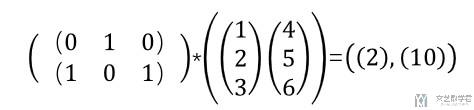1. # torch.bmm测试-这是矩阵乘法
2. x_test = torch.tensor([[[0,1,0]],[[1,0,1]]])
3. y_test = torch.tensor([[,,],[,,]])
4. print(x_test.shape,y_test.shape)
5. print(torch.bmm(x_test,y_test))
6. """
7. torch.Size([2, 1, 3]) torch.Size([2, 3, 1])
8. tensor([[[ 2]],
9.         []])
10. """

## Pytorch生成随机数

### 生成服从正态分布的随机数

1. a = torch.from_numpy(np.array([[1,2,3],[4,5,6]]))
2. torch.randn(*a.size())
3. """
4. tensor([[-2.4163, -0.9349, -0.1467],
5.         [-1.4294, -0.3001,  0.7097]])
6. """

### 生成整数随机数

1. torch.ones([3, 3], dtype=torch.float64).random_(10, 20)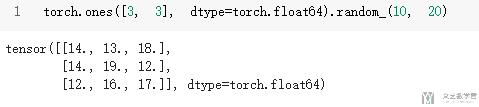## Softmax的一些用法

### Softmax的dim的一些说明

1. # Softmax测试
2. x_test = torch.tensor([[[0,1,0]],[[1,1,1]]]).float()
3. print(x_test.shape)
4. F.softmax(x_test,dim=2)
5. """
6. torch.Size([2, 1, 3])
7. tensor([[[0.2119, 0.5761, 0.2119]],
8.         [[0.3333, 0.3333, 0.3333]]])
9. """

### Softmax与LogSoftmax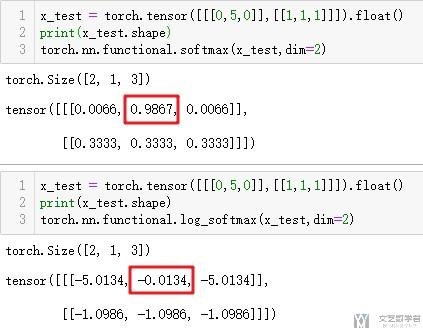## weight clip的用法

1. a = torch.tensor([-1.1, 0.8, 1.2, -0.6, 0.9])
2. a.data.clamp_(-1, 1) # 这个是可以改变原始的a的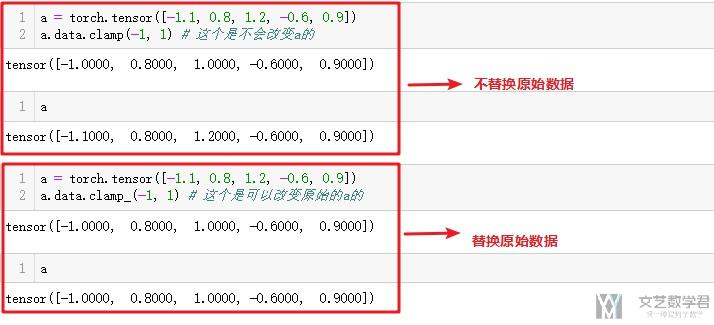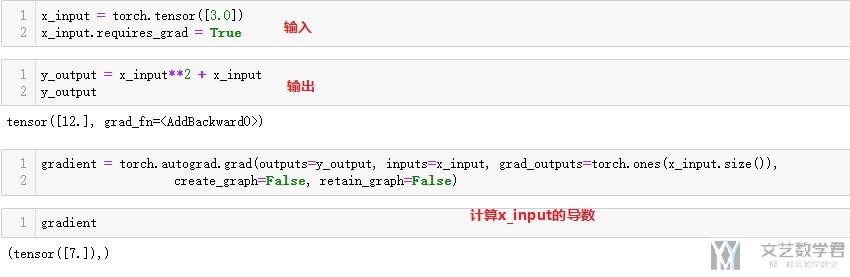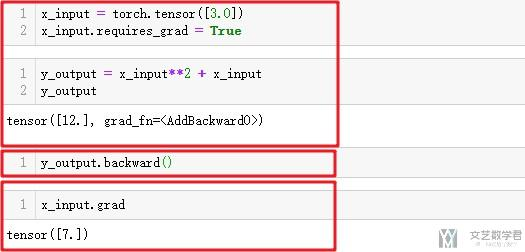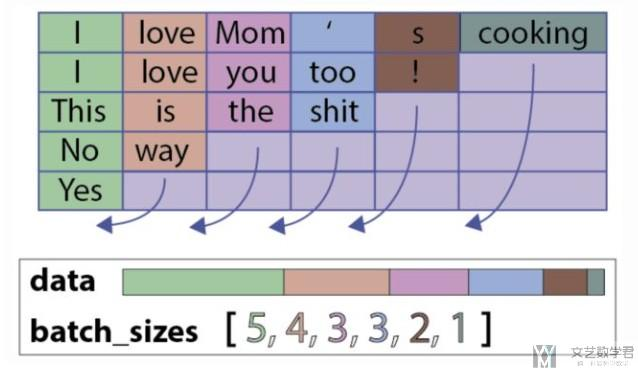1. # SORT YOUR TENSORS BY LENGTH!(按长度进行排序)
2. seq_lengths, perm_idx = seq_lengths.sort(0, descending=True)
3. seq_tensor = seq_tensor[perm_idx]
4. # utils.rnn lets you give (B,L,D) tensors where B is the batch size, L is the maxlength, if you use batch_first=True
5. # Otherwise, give (L,B,D) tensors
6. seq_tensor = seq_tensor.transpose(0,1) # (B,L,D) -> (L,B,D)
7. # embed your sequences(embedding)
8. seq_tensor = embed(seq_tensor)
9. # pack them up nicely
11. # throw them through your LSTM (remember to give batch_first=True here if you packed with it)
12. packed_output, (ht, ct) = lstm(packed_input)
13. # unpack your output if required
15. print (output)
16. # Or if you just want the final hidden state?
17. print (ht[-1])

1. class ClickSingleGRU(nn.Module):
2.     def __init__(self, vocab_size, embedding_dim, hidden_units, batch_sz, output_size, layers=2, Drop_switch=False):
3.         super(ClickSingleGRU, self).__init__()
4.         self.Drop_switch = Drop_switch # 是否使用dropout
5.         self.vocab_size = vocab_size # 总的单词的个数
6.         self.embedding_dim = embedding_dim # embedding之后的元素个数
7.         self.hidden_units = hidden_units # GRU中的hidden units的个数
8.         self.batch_sz = batch_sz
9.         self.output_size = output_size
10.         self.num_layers = layers # GRU的layers
11.         # layers
12.         self.embedding = nn.Embedding(self.vocab_size, self.embedding_dim) # 可以将标号转为向量
13.         self.dropout = nn.Dropout(0.5)
14.         self.gru = nn.GRU(self.embedding_dim, self.hidden_units, num_layers=self.num_layers, batch_first = True, bidirectional=True, dropout=0.5)
15.         self.fc = nn.Linear(self.hidden_units*2, self.output_size)
16.         self.batchNorm = nn.BatchNorm1d(self.hidden_units*2)
17.     def init_hidden(self):
18.         # 使用了双向RNN, 所以num_layer*2
19.         return torch.zeros((self.num_layers*2, self.batch_sz, self.hidden_units)).to(device1)
20.     def forward(self, x, x_len):
21.         x = x.to(device1)
22.         # 按照顺序进行排序
23.         _, perm_idx = x_len.sort(0, descending=True)
24.         x = x[perm_idx] # 对样本x重新排序
25.         x_len = x_len[perm_idx] # 对长度x重新排序
26.         # 进行正向传播
27.         self.batch_sz = x.size(0)
28.         x = self.embedding(x)
29.         # print(x.shape)
30.         # print(x_len)
31.         x = pack_padded_sequence(x, x_len, batch_first=True)
32.         self.hidden = self.init_hidden()
33.         output, self.hidden = self.gru(x, self.hidden)
34.         output, _ = pad_packed_sequence(output, batch_first=True)
35.         # print(output.shape)
36.         # 因为是 batch*seq*output, 所以要取最后一个seq
37.         output = output[:,-1,:]
38.         # print(output.shape)
39.         if self.Drop_switch:
40.             output = self.dropout(output)
41.         output = self.batchNorm(output)
42.         output = self.fc(output)
43.         # print(output.shape)
44.         # 对输出重新排序, 这个需要与最后的label对应上
45.         output = output[perm_idx]
46.         # print(output.shape)
47.         return output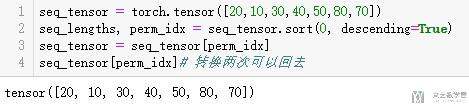• 微信公众号
• 关注微信公众号
•• QQ群
• 我们的QQ群号
•• 本文由 发表于 2019年6月14日07:59:21
• 转载请务必保留本文链接：https://mathpretty.com/10594.html